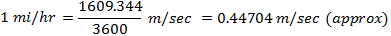1. Worksheets>
2. Math>
3. Solve>
4. Speed>
5. Conversion 6

# How to convert miles/hr into m/sec

1 mile = 1609.344 m (approx)

1 hour = 3600 secTo convert miles/hr into m/sec, multiply the number by 0.44704 and then round off the answer to the required decimal places.

Solved Example 1:

Convert 30 miles/hr into m/sec. Round off the answer to the nearest whole numbers.

Solution:

30 miles/hr

Step 1:

Multiply 30 by 0.44704

We have 30 * 0.44704 = 13.4112

Step 2:

Round off to the nearest whole number

13.4112 = 13 (approx)

30 miles/hr = 13 m/sec (approx)

Try yourself: Practice Sheet 1

Solved Example 2:

Convert 100 miles/hr into m/sec. Round off the answer to 2 decimal places.

Solution:

100 miles/hr

Step 1:

Multiply 100 by 0.44704

We have 100 * 0.44704 = 44.704

Step 2:

Round off to 2 decimal places

44.704 = 44.7 (approx)